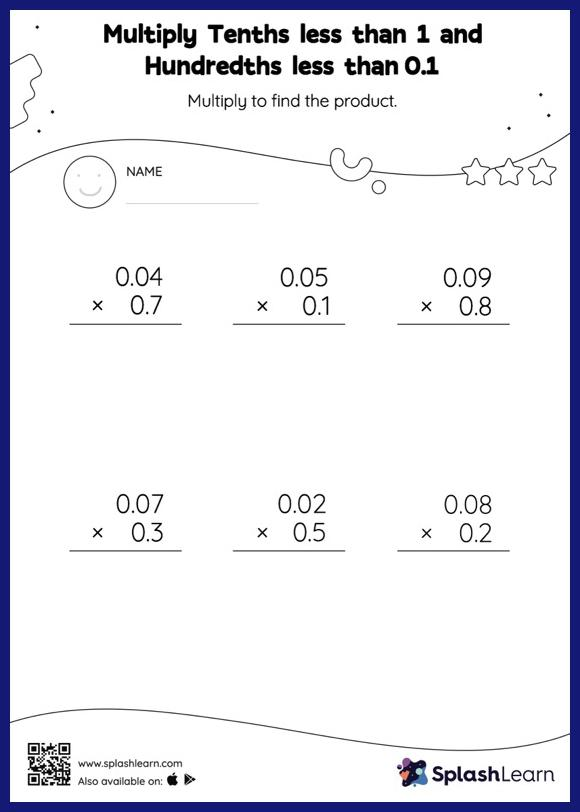# Multiply Tenths less than 1 and Hundredths less than 0.1: Vertical Multiplication Worksheet

Home > Multiply Tenths less than 1 and Hundredths less than 0.1: Vertical MultiplicationThis worksheet gives students an opportunity to apply the concepts of multiplication to multiply tenths less than 1 and hundredths less than 0.1. Students first multiply as though there is no decimal when multiplying two decimals. They then add the same number of digits after the decimal point in the product as there are decimal digits in each number. This multiply tenths less than 1 and hundredths less than 0.1 Worksheet gives students a thorough practice of this concept.